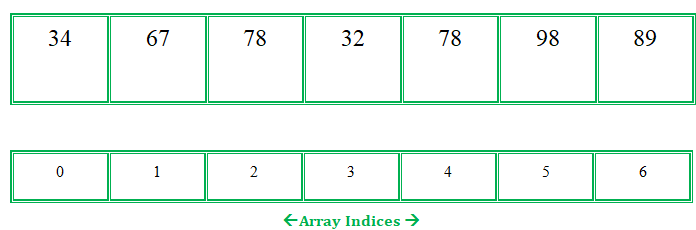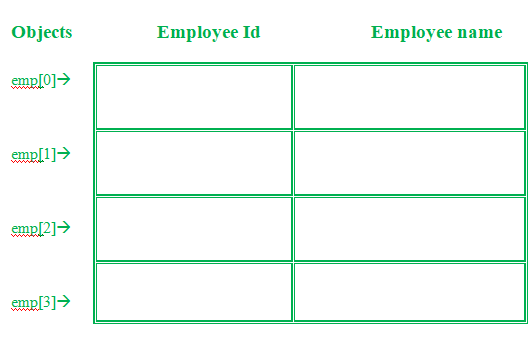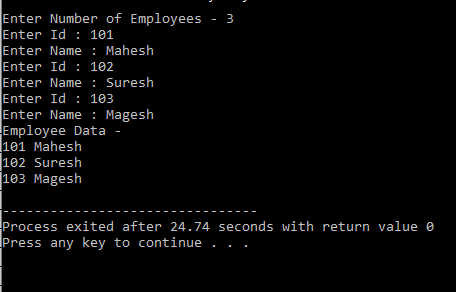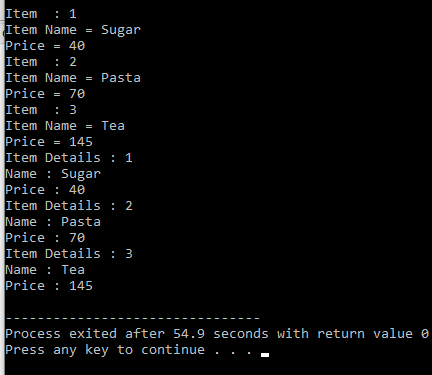GeeksforGeeks App
Open AppBrowser
Continue

# Array of Objects in C++ with Examples

An array in C/C++ or be it in any programming language is a collection of similar data items stored at contiguous memory locations and elements can be accessed randomly using indices of an array.  They can be used to store the collection of primitive data types such as int, float, double, char, etc of any particular type. To add to it, an array in C/C++ can store derived data types such as structures, pointers, etc. Given below is the picture representation of an array.

Example:
Let’s consider an example of taking random integers from the user.Array

Array of Objects

When a class is defined, only the specification for the object is defined; no memory or storage is allocated. To use the data and access functions defined in the class, you need to create objects.

Syntax:

`ClassName ObjectName[number of objects];`

The Array of Objects stores objects. An array of a class type is also known as an array of objects.

Example#1:
Storing more than one Employee data. Let’s assume there is an array of objects for storing employee data emp.Below is the C++ program for storing data of one Employee:

## C++

 `// C++ program to implement``// the above approach``#include``using` `namespace` `std;` `class` `Employee``{``  ``int` `id;``  ``char` `name;``  ``public``:``  ``void` `getdata();``//Declaration of function``  ``void` `putdata();``//Declaration of function``};``void` `Employee::getdata(){``//Defining of function``  ``cout<<``"Enter Id : "``;``  ``cin>>id;``  ``cout<<``"Enter Name : "``;``  ``cin>>name;``}``void` `Employee::putdata(){``//Defining of function``  ``cout<

Let’s understand the above example –

• In the above example, a class named Employee with id and name is being considered.
• The two functions are declared-
• getdata(): Taking user input for id and name.
• putdata(): Showing the data on the console screen.

This program can take the data of only one Employee. What if there is a requirement to add data of more than one Employee. Here comes the answer Array of Objects. An array of objects can be used if there is a need to store data of more than one employee. Below is the C++ program to implement the above approach-

## C++

 `// C++ program to implement``// the above approach``#include``using` `namespace` `std;` `class` `Employee``{``  ``int` `id;``  ``char` `name;``  ``public``:``  ` `  ``// Declaration of function``  ``void` `getdata();``  ` `  ``// Declaration of function``  ``void` `putdata();``};` `// Defining the function outside``// the class``void` `Employee::getdata()``{``  ``cout << ``"Enter Id : "``;``  ``cin >> id;``  ``cout << ``"Enter Name : "``;``  ``cin >> name;``}` `// Defining the function outside``// the class``void` `Employee::putdata()``{``  ``cout << id << ``" "``;``  ``cout << name << ``" "``;``  ``cout << endl;``}` `// Driver code``int` `main()``{``  ``// This is an array of objects having``  ``// maximum limit of 30 Employees``  ``Employee emp;``  ``int` `n, i;``  ``cout << ``"Enter Number of Employees - "``;``  ``cin >> n;``  ` `  ``// Accessing the function``  ``for``(i = 0; i < n; i++)``    ``emp[i].getdata();``  ` `  ``cout << ``"Employee Data - "` `<< endl;``  ` `  ``// Accessing the function``  ``for``(i = 0; i < n; i++)``    ``emp[i].putdata();``}`

Output:Explanation:
In this example, more than one Employee’s details with an Employee id and name can be stored.

• Employee emp – This is an array of objects having a maximum limit of 30 Employees.
• Two for loops are being used-
• First one to take the input from user by calling emp[i].getdata() function.
• Second one to print the data of Employee by calling the function emp[i].putdata() function.

Example#2:

## C++

 `// C++ program to implement``// the above approach``#include``using` `namespace` `std;``class` `item``{``  ``char` `name;``  ``int` `price;``  ``public``:``  ``void` `getitem();``  ``void` `printitem();``};`` ` `// Function to get item details``void` `item::getitem()``{``  ``cout << ``"Item Name = "``;``  ``cin >> name;``  ``cout << ``"Price = "``;``  ``cin >> price;   ``}` `// Function to print item``// details``void` `item ::printitem()``{``  ``cout << ``"Name : "` `<< name <<``          ``"\n"``;``  ``cout << ``"Price : "` `<< price <<``          ``"\n"``;``}` `const` `int` `size = 3;` `// Driver code``int` `main()``{``  ``item t[size];``  ``for``(``int` `i = 0; i < size; i++)``  ``{``    ``cout << ``"Item  : "` `<<``            ``(i + 1) << ``"\n"``;``    ``t[i].getitem();``  ``}``  ` `  ``for``(``int` `i = 0; i < size; i++)``  ``{``    ``cout << ``"Item Details : "` `<<``             ``(i + 1) << ``"\n"``;``    ``t[i].printitem();``  ``}``}`

Output: